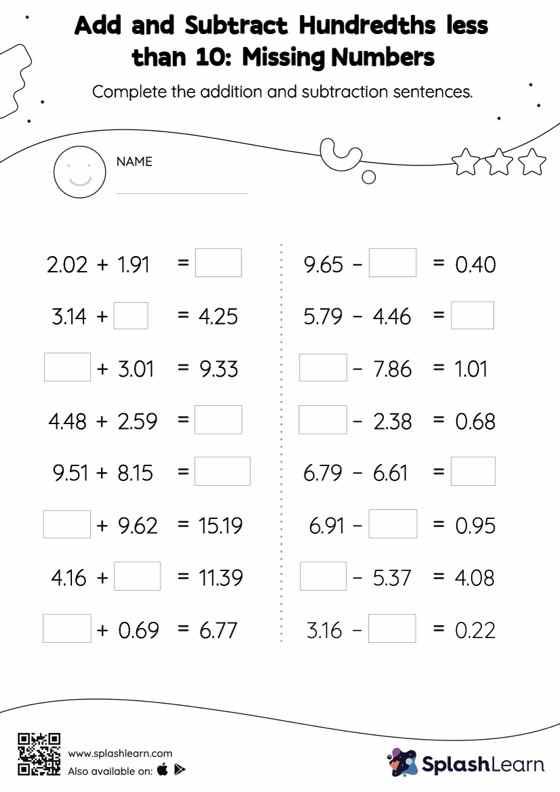# Add and Subtract Hundredths less than 10: Missing Numbers Worksheet

Home > Add and Subtract Hundredths less than 10: Missing NumbersAdd and subtract hundredths less than 10 worksheet is a great way to help learners become fluent with the concept of addition and subtraction. Students align the decimal points and use zero as space holders to add and subtract decimals. Then they use the relationship between addition and subtraction to find the missing number in add and subtract hundredths less than 10 worksheet.